# Measuring Angles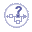The following section describes:

## Exact Angles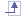The Measure Between command lets you measure exact angles between the following geometrical entities that have (at least) one common point.

#### Important

• Angle calculation between two curves or between one curve and one line can only be performed in exact mode. In the same way, selecting the intersection points between two curves or between one line and one curve is possible only in exact mode.

• Note that even if the exact mode is selected, the tolerance for angle calculation is 10e-4 rad (tolerance value: 0.005 deg)

#### Angle measurement between a plane and a curve

In exact mode, angle calculation between a plane and a curve (the curve intersects the plane) result is the angle between the direction of the plane (displayed in red) and the tangent of the curve at the intersection point (displayed in blue).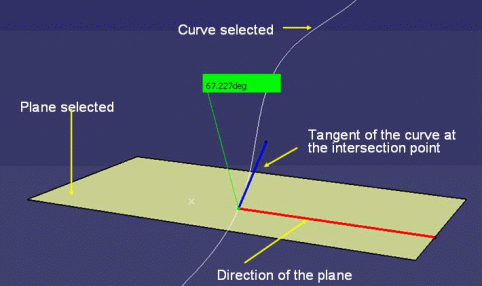#### Angle measurement between two curves (or between a line and a curve)

Two lines (even if not in the same plane):

A line and a  curve: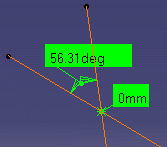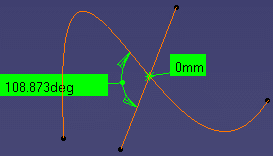Two curves: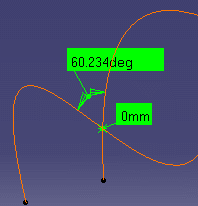Notes:

• In the above three cases, if entities intersect more than once, the measure is made at the point of intersection nearest the point at which selection 1 is made.

• The angle measurement between a curve and a plane or between a curve and a line (even if we select them using the infinite mode) is performed only if the curve intersects the plane or the line.

#### Example below:

The spline intersects the plane 1, in this case: the angle is measured.

The spline does not intersect the plane 2, in this case only the distance is measured.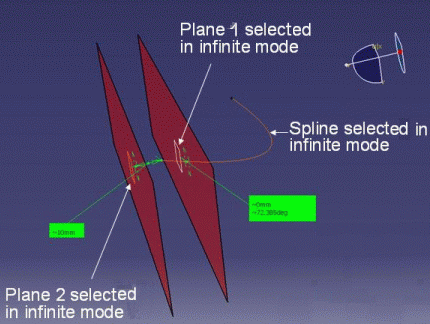A curve and a surface: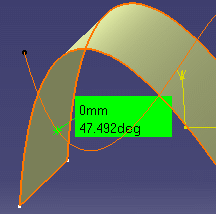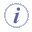Note: If the curve and surface intersect more than once, the measure is made at the point of intersection nearest the point of the selection on the curve.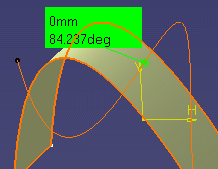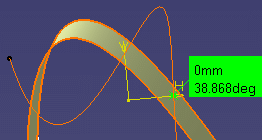A line and a surface:

A line and a plane: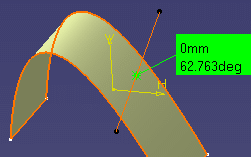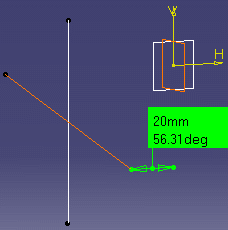Two surfaces: You can also measure the angle between two surfaces provided both surfaces are planar.

## Complementary AnglesYou can obtain the complementary angle (360° - the initial angle measured) when measuring between two curves: drag the angle line to show the complementary angle.Note: The dialog box and knowledge parameters are refreshed. The value of the complementary angle is stored along with the measure.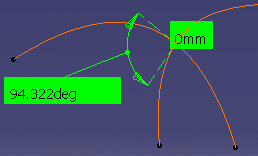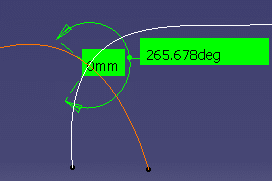For any two geometrical entities that meet, the choice of measurement between a given angle or its complementary angle is performed with respect to where you select the entities in the 3D area: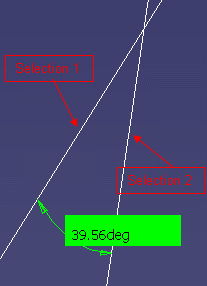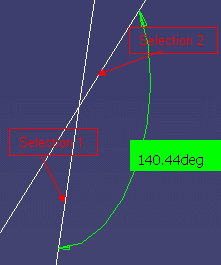Notes:

• If you select entities in the specification tree, the measured angle is given arbitrarily (A or 180 degrees - A)

• The orientation of the curves or lines is not taken into account